Click to Chat

1800-1023-196

+91-120-4616500

CART 0

• 0

MY CART (5)

Use Coupon: CART20 and get 20% off on all online Study Material

ITEM
DETAILS
MRP
DISCOUNT
FINAL PRICE
Total Price: Rs.

There are no items in this cart.
Continue Shopping• Complete JEE Main/Advanced Course and Test Series
• OFFERED PRICE: Rs. 15,900
• View Details

```Electric Charge

Table of Content

What is Electric Charge?

Another Definition of Electric Charge

What is the definition of charge in daily life?

How Charge Originate?

What is SI unit of Charge?

What is Elementary Charge?

How many types of charge exist in nature?

What are the Properties of Electric Charge?

How Electrons Come out from the Neutral Object?

How can we charge an object?

How Electric Field can generate from Charge?

What is Current?

Another Definition of Electric Current

What is Formula of Current?

What is SI Unit of Current?

What is Dimensional Formula of Current?

What is Electric Charge?

Electric Charge is a physical property of matter due to which a matter experience a force on another charge matter when placed in electromagnetic field (electric or magnetic field).

Another Definition of Electric Charge

According to Franklin electric charge is used for electrostatic interaction. For Example: A body can interact with another body only if charge is present in that body.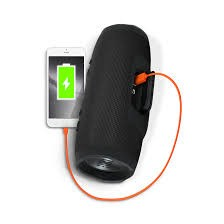What is the definition of charge in daily life?

In daily life we use the term charge many times. For Example: charging a phone. We can charge an object when we apply voltage to it.

Q= CV

Q  = Charge, C = Capacitance, V= Voltage.

How Charge Originate?

It was assumed that origin of charge start from atomic structure. Let us consider a atom having size 10 -10 meter. Inside the atom nucleus in present and size of nucleus is 10 -15 meter. Electrons revolve around the nucleus. As we know that symbol of proton is (P + ) so charge on proton is + and symbol of electron is (e – ) and charge on electron is – so from sign convention we can tell that the object is positively charged or negatively.In the above figure we can see that how charge originate from the atomic structure. From the atom electrons and protons originate.

What is SI unit of Charge?

SI unit of charge is Coulomb (C).

What is Elementary Charge?

Elementary Charge is the magnitude of charge carried by single electron or proton. The charge on electron is negative and it is denoted by (-e) while the charge on proton is positive and it is denoted by (+e).

The charge on single proton is (+ 1.6 * 10 -19 C ) while the charge on single electron is (- 1.6 * 10 -19 C).

How many types of charge exist in nature?

There are two types of charge exists in nature:

Positive Charge
Negative Charge

“Same charge repel each other and opposite charge attract each other”

In the diagram given below we can see that same charge repel each other and opposite charge attract each other.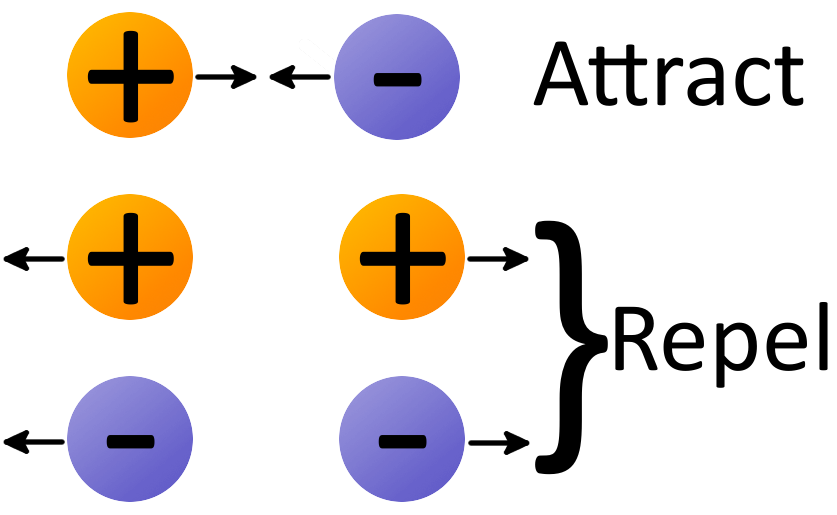Note : In case of neutral charge number of positive charge is equal to the number of negative charge but neutral charge is not considered the type of charge. There are only two types of charge exist. For Example: Positive and Negative.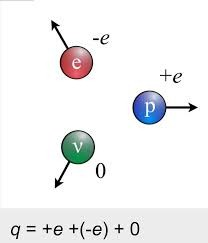What are the Properties of Electric Charge?

Electric Charge is conserved, it can neither be created nor be destroyed in an isolated system.

The SI unit of charge is Coulomb (C).

The object becomes positively charge if it gives some charge to another object.

The object which accept the charge from another body become Negatively Charged.

Charge is quantized in nature.

How Electrons Come out from the Neutral Object?

Let us consider a neutral object (equal number of proton and electron). In neutral object each electron is attracted to each proton (because number of positive charge is equal to number of negative charge). The force we are applying  to pull electrons from the object is equal to the force which is pulling  back electrons to the charged object because amount of positive  charge is equal to the negative charge, so both forces cancel out with each other. So we have to do some external work to pull the electrons from the object.

There are various method to pull the electrons from the surface.

How electrons can pull from the object with the help of battery: Battery consist  two terminals positive and negative. On applying the external voltage electrons are attracted towards the positive terminal and electron can pull out of the object.

Another way to pull electron is hit an object with high energy so that electrons come out from the object, that energy is more than the energy which are pulling electrons back to the metal surface.

How can we charge an object?

There are three methods to charge an object:

Charge by induction method

Charge by conduction method

Charge by friction method

How Electric Field can generate from Charge?

Electric Field  Generate when charge is in rest while the moving charge produce magnetic field.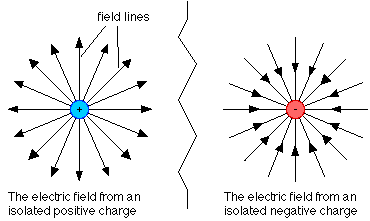In case of positive charge direction of electric field is away from the charge while in case of negative charge direction of electric field is towards the charge as shown in the figure.

What is Current?

Current may be defined as charge flowing through a closed circuit per unit time.

Another Definition of Electric Current

A current is said to be one Ampere if charge of one Coulomb flows  through a point in one Second.

I = q/t

Image of Electric Current in daily life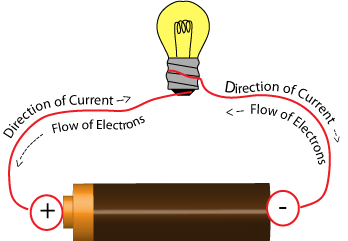In above figure, current is flow in the circuit when we apply the voltage to the circuit the charge generates and electrons flows in the circuit. The direction of current is opposite to the direction of flow of electrons.

What is Formula of Current?

I = q/t

Where,  I is the current flowing in the circuit

q is the charge flowing in the circuit

t is the time.

What is SI Unit of Current?

SI unit of current is Ampere.

What is Dimensional Formula of Current?

Dimensional formula of current is [ CS-1 ].

Q1. What is the definition of charge?

Sol. A charge is said to be one coulomb if one Ampere current flows in a circuit in one second.

Q2. What are positive and negative charge?

Sol.  A neutral atom becomes an ion if it loose or gain electron or proton. As we know that neutral atom don’t have any charge it becomes positive charge if it loose electron and it becomes negative charge if it gain electron.

Q3. What is the unit for measurement of charge?

Sol. The unit for measurement of charge is coulomb.

Q4. What is an electric current?

Sol. Electric current is the ratio of charge per unit time.

Q5. Why do two electrons repel each other?

Sol. Two electrons repel each other because both the electrons have same charge. For Example: Negative and we know that same charge repel each other.

Watch this Video for more reference

```### Course Features

• 728 Video Lectures
• Revision Notes
• Previous Year Papers
• Mind Map
• Study Planner
• NCERT Solutions
• Discussion Forum
• Test paper with Video Solution